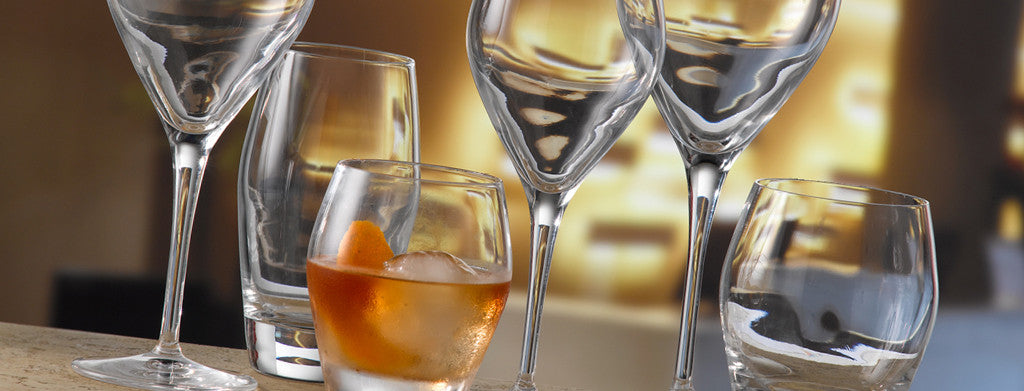Shopping Cart

### Refine

View all

#### Price# Glassware£0.94 each (ex VAT)£4.47 each (ex VAT)£0.25 each (ex VAT)£4.06 each (ex VAT)£3.42 each (ex VAT)£4.22 each (ex VAT)£2.64 each (ex VAT)£7.37 each (ex VAT)£14.85 each (ex VAT)£3.42 each (ex VAT)£3.33 each (ex VAT)£0.25 each (ex VAT)£6.28 each (ex VAT)£1.72 each (ex VAT)£5.28 each (ex VAT)£4.05 each (ex VAT)£2.68 each (ex VAT)£4.23 each (ex VAT)£9.54 each (ex VAT)£2.67 each (ex VAT)£13.42 each (ex VAT)£7.37 each (ex VAT)£20.30 each (ex VAT)£4.23 each (ex VAT)£26.50 each (ex VAT)£22.67 each (ex VAT)£2.80 each (ex VAT)£5.69 each (ex VAT)£22.53 each (ex VAT)£17.54 each (ex VAT)£4.40 each (ex VAT)£4.33 each (ex VAT)£0.67 each (ex VAT)£1.98 each (ex VAT)£3.56 each (ex VAT)£30.00 each (ex VAT)£4.65 each (ex VAT)£3.42 each (ex VAT)£2.37 each (ex VAT)£2.48 each (ex VAT)£1.98 each (ex VAT)£2.11 each (ex VAT)£6.76 each (ex VAT)£6.80 each (ex VAT)£3.66 each (ex VAT)£3.37 each (ex VAT)£3.92 each (ex VAT)£3.37 each (ex VAT)